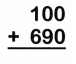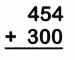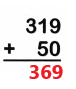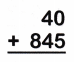# McGraw Hill Math Grade 2 Chapter 5 Lesson 7 Answer Key Use Mental Math to Add 10s or 100s to a Number

Practice questions available in McGraw Hill Math Grade 2 Answer Key PDF Chapter 5 Lesson 7 Use Mental Math to Add 10s or 100s to a Number will engage students and is a great way of informal assessment.

## McGraw-Hill Math Grade 2 Answer Key Chapter 5 Lesson 7 Use Mental Math to Add 10s or 100s to a Number

Use mental math to add. Write the sum.

Question 1.
527 + 10 = 537

Question 2.
366 + 30 = ___
396

Explanation:
60 + 30 = 90
So, 366 + 30 = 396

Question 3.
568 + 400 = _____
968

Explanation:
500 + 400 = 900
So, 568 + 400 = 968.

Question 4.
263 + 200 = ___
463

Explanation:
200 + 200 = 400
So, 263 + 200 = 463.

Question 5.Explanation:
The sum of 100 and 690 is 790.

Question 6.Explanation:
The sum of 454 and 300 is 754.

Question 7.Explanation:
The sum of 319 and 50 is 369.

Question 8.